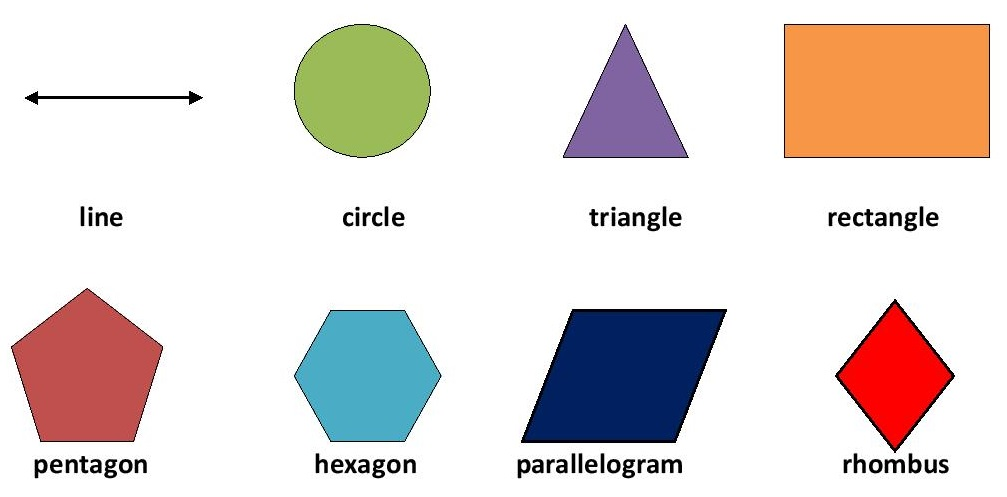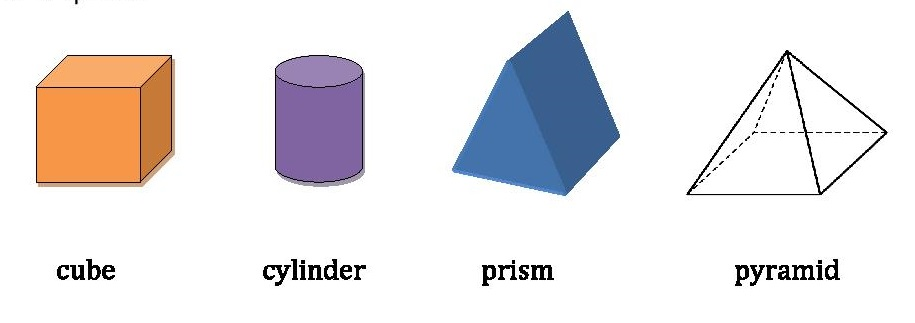Top

# GCSE Geometric

### INTRODUCTION

Geometry is a branch of mathematics that deals with shapes and their properties.The properties include finding the area, perimeter,volume, length, width, height, angles and so on.It can be divided into two:

• Plane Geometry
• Solid geometry

PLANE GEOMETRY:

It studies about flat shapes like lines, circles, triangles and polygons that can be drawn on a piece of paper.It includes both one-dimensional and two- dimensional figures.SOLID GEOMETRY:

It studies about three-dimensional objects like cube, prism, pyramid, cylinder and sphere.Properties:

Area: It is the amount of two-dimensional space occupied by a shape.

Perimeter: It is the total length of the outer boundary of an enclosed figure.

Volume: It is the amount of space inside a three-dimensional object.

Lateral surface area: It is the sum of surface areas of all sides except the top and bottom face of solid.

Total surface area: It is the sum of surface areas of all sides of a solid figure.